# Primitive recursive function

A function from natural numbers to natural numbers which can be obtained from the initial functionsby a finite number of the operations of composition and primitive recursion.

Since the initial functions are computable and the operators of superposition and primitive recursion preserve computability, the set of all primitive recursive functions is a subclass of the class of all computable functions (cf. Computable function). Every primitive recursive function is specified by a description of its construction from the initial functions (a primitive recursive description); hence the class of primitive recursive functions is countable. Practically all arithmetic functions used in mathematics for some concrete reason are primitive recursive functions; e.g.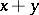,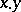,,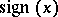,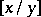(the remainder from division ofby),(the prime number with index), etc.

A relationon natural numbers is called a primitive recursive relation if the function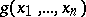, equal to 1 if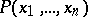is true and 0 ifis false, is primitive recursive. One says that the relationhas been obtained from a relationby means of a bounded quantifier ifor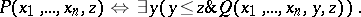The class of primitive recursive relations is closed under the application of logical connectives (including negation) and bounded quantifiers.

Suppose that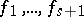are-place primitive recursive functions, and letbe primitive recursive relations such that for any set of argument values at most one of them is true. Then the function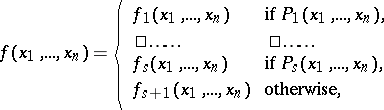(*)

is primitive recursive.

One says that the function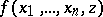has been obtained from an everywhere-defined function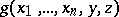by means of the bounded minimization operator ifis equal to the minimalsuch thatand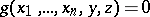, and is equal to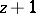otherwise. The class of primitive recursive functions is closed under bounded minimization operators.

A function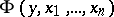is called universal for the class of-place primitive recursive functions if for each such function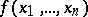there is a natural numbersuch thatThere exists a universal function for every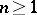, but it need not be primitive recursive.

Every recursively enumerable set is the range of values of a primitive recursive function; every recursively enumerable relation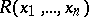can be represented as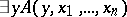, whereis a primitive recursive relation. Every primitive recursive function can be represented in formal arithmetic (cf. Arithmetic, formal); i.e. for each primitive recursive functionthere is an arithmetical formula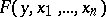such that for all natural numbers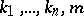, the formula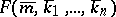is derivable in formal arithmetic if, while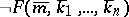is derivable if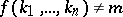. (Here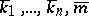are arithmetical terms reflecting the natural numbersin formal arithmetic.) This fact takes a central position in the proof of the incompleteness of formal arithmetic (cf. ).